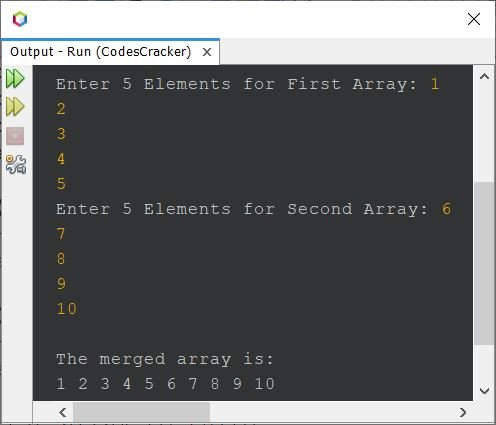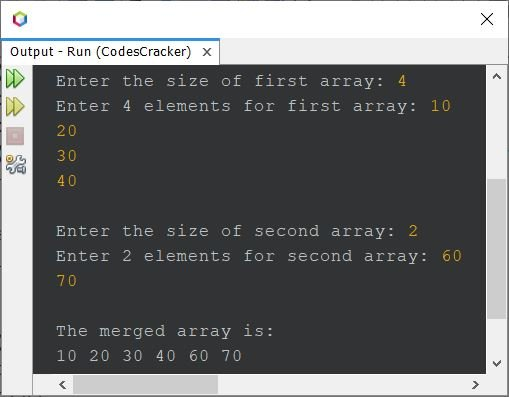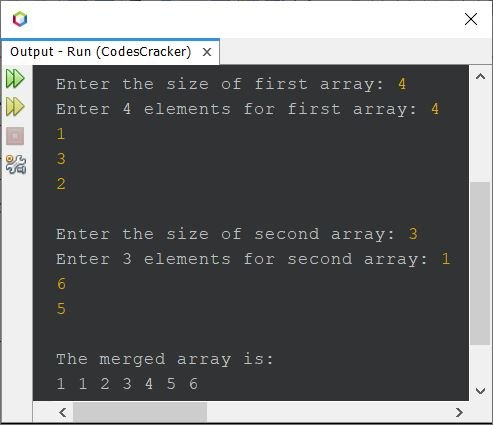# Java Program to Merge Two Arrays

This article covers multiple programs in Java that merges two given arrays into the third array. Here are the list of programs covered in this article:

• Merge two arrays in Java - basic version
• Merge two arrays in Java - complete version
• Merge two arrays into the third array and sort in ascending order
• Merge two arrays in descending order

## Merge Two Arrays in Java - Basic Version

The question is, write a Java program to merge two arrays. Both the array must be received by user at run-time of the program. The program given below is its answer:

```import java.util.Scanner;

public class CodesCracker
{
public static void main(String[] args)
{
int i, k=0;
int[] a = new int;
int[] b = new int;
int[] c = new int;
Scanner scan = new Scanner(System.in);

System.out.print("Enter 5 Elements for First Array: ");
for(i=0; i<5; i++)
a[i] = scan.nextInt();

System.out.print("Enter 5 Elements for Second Array: ");
for(i=0; i<5; i++)
b[i] = scan.nextInt();

// copying the first array to the third array
for(i=0; i<5; i++, k++)
c[k] = a[i];

// copying the second array to the third array
for(i=0; i<5; i++, k++)
c[k] = b[i];

System.out.println("\nThe merged array is: ");
for(i=0; i<10; i++)
System.out.print(c[i]+ " ");
}
}```

The snapshot given below shows the sample run of above program with user input 1, 2, 3, 4, 5 as five elements for first, whereas 6, 7, 8, 9, 10 as five elements for the second array:## Merge Two Arrays in Java - Complete Version

This is the modified version of previous program, allows user to define the size of both the arrays too, along with its elements, to merge two arrays of given size:

```import java.util.Scanner;

public class CodesCracker
{
public static void main(String[] args)
{
int i, k=0;
int[] merge = new int;
Scanner scan = new Scanner(System.in);

System.out.print("Enter the size of first array: ");
int x = scan.nextInt();
int[] a = new int[x];
System.out.print("Enter " +x+ " elements for first array: ");
for(i=0; i<x; i++)
{
a[i] = scan.nextInt();
merge[k] = a[i];
k++;
}
System.out.print("\nEnter the size of second array: ");
int y = scan.nextInt();
int[] b = new int[y];
System.out.print("Enter " +y+ " elements for second array: ");
for(i=0; i<y; i++)
{
b[i] = scan.nextInt();
merge[k] = b[i];
k++;
}

System.out.println("\nThe merged array is: ");
for(i=0; i<k; i++)
System.out.print(merge[i]+ " ");
}
}```

Here is its sample run with user inputs, 4 as size, 10, 20, 30, 40 as four elements for the first array, and 2 as size, 60, 70 as two elements for the second array:## Java Merge Two Arrays and Sort in Ascending Order

This program merges two given arrays into the third array, and then sort the merged array in ascending order, before print.

```import java.util.Scanner;

public class CodesCracker
{
public static void main(String[] args)
{
int i, j;
Scanner scan = new Scanner(System.in);

System.out.print("Enter the size of first array: ");
int x = scan.nextInt();
int[] a = new int[x];
System.out.print("Enter " +x+ " elements for first array: ");
for(i=0; i<x; i++)
a[i] = scan.nextInt();

System.out.print("\nEnter the size of second array: ");
int y = scan.nextInt();
int[] b = new int[y];
System.out.print("Enter " +y+ " elements for second array: ");
for(i=0; i<y; i++)
b[i] = scan.nextInt();

// merging both the entered arrays into the third array
int[] merge = new int[x+y];
for(i=0; i<x; i++)
merge[i] = a[i];
for(j=0; j<y; j++)
merge[i++] = b[j];

// sorting the merged array
int k = i;
for(i=0; i<(k-1); i++)
{
for(j=0; j<(k-1); j++)
{
if(merge[j]>merge[j+1])
{
int temp = merge[j];
merge[j] = merge[j+1];
merge[j+1] = temp;
}
}
}

// printing the sorted merged array
System.out.println("\nThe merged array is: ");
for(i=0; i<k; i++)
System.out.print(merge[i]+ " ");
}
}```

The sample run with user inputs 4 as size, 4, 1, 3, 2 as four elements of first, and 3 as size, 1, 6, 5 as three elements of second array, is shown in the snapshot given below:Here are the list of sorting technique, you can use in above program to sort the array after merge:

## Java Merge Two Arrays in Descending Order

To merge two arrays into third array and sort in descending order, the program will exactly be the same as of previous program, except a single sign (>) character. That is, from the above program, change the following code:

`if(merge[j]>merge[j+1])`

with the code given below:

`if(merge[j]<merge[j+1])`

Just a matter of sign, the whole program gets changed (reversed).

#### Same Program in Other Languages

Java Online Test

« Previous Program Next Program »